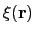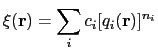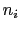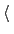Next: Colvars as scripted functions Up: Collective variable components (basis Previous: Advanced usage and special   Contents   Index

## Linear and polynomial combinations of components

To extend the set of possible definitions of colvars, multiple componentscan be summed with the formula:(13.13)

where each component appears with a unique coefficient(1.0 by default) the positive integer exponent(1 by default).

Any set of components can be combined within a colvar, provided that they return the same type of values (scalar, unit vector, vector, or quaternion). By default, the colvar is the sum of its components. Linear or polynomial combinations (following equation (13.14)) can be obtained by setting the following parameters, which are common to all components:

• componentCoeffCoefficient of this component in the colvarContext: any component
Acceptable values: decimal
Default value: 1.0
Description: Defines the coefficient by which this component is multiplied (after being raised to componentExp) before being added to the sum.

• componentExpExponent of this component in the colvarContext: any component
Acceptable values: integer
Default value: 1
Description: Defines the power at which the value of this component is raised before being added to the sum. When this exponent is different than 1 (non-linear sum), total forces and the Jacobian force are not available, making the colvar unsuitable for ABF calculations.

Example: To define the average of a colvar across different parts of the system, simply define within the same colvar block a series of components of the same type (applied to different atom groups), and assign to each component a componentCoeff of.Next: Colvars as scripted functions Up: Collective variable components (basis Previous: Advanced usage and special   Contents   Index
vmd@ks.uiuc.edu